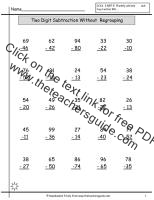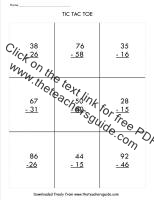Two Digit SubtractionCount Back to Subtract Ones and Tens Worksheet

Common Core State Standards: CCSS 2.NBT.5 Fluently add and subtract within 100….
Count back to subtract ones and tens from two digit numbers.Subtracting Tens Worksheet

Common Core State Standards: CCSS 2.NBT.5 Fluently add and subtract within 100….
Count back to subtract tens from two digit numbers.  Hundred chart included.  25 problemsSubtract Tens from Tens Worksheet.

Common Core State Standards: CCSS 2.NBT.5 Fluently add and subtract within 100….
Count back to subtract tens from tens.  25 problems.Subtract Tens from Two Digit Numbers Worksheet

Common Core State Standards: CCSS 2.NBT.5 Fluently add and subtract within 100….
Count back to subtract tens from two digit numbers.  25 problems.Subtracting One Digit Numbers from Two Digit Numbers Worksheet 2, 3

Common Core State Standards: CCSS 2.NBT.5 Fluently add and subtract within 100….
Subtract one digit numbers from two digit numbers.  25 problems.Two Digit Subtraction Without Regrouping Worksheet 2, 3,    4

Common Core State Standards: CCSS 2.NBT.5 Fluently add and subtract within 100….
Subtract two digit numbers from two digit numbers without regrouping.Two Digit Subtraction With Regrouping Worksheet With Place Value Chart

Subtraction with regrouping with a place value chart to draw place value discs.Two Digit Subtraction With Regrouping Worksheet 2, 3, 4

Common Core State Standards: CCSS 2.NBT.5 Fluently add and subtract within 100….
Subtract two digit numbers from two digit numbers with regrouping.Two Digit Subtraction With Regrouping Tic Tac Toe

Subtract two digit numbers from two digit numbers tic tac toe.Mixed Subtraction Review

Mixed subtraction review.